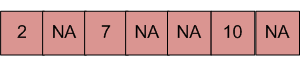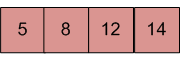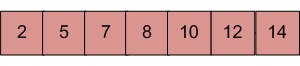GFG App
Open AppBrowser
Continue

# Merge an array of size n into another array of size m+n

There are two sorted arrays. First one is of size m+n containing only m elements. Another one is of size n and contains n elements. Merge these two arrays into the first array of size m+n such that the output is sorted.
Input: array with m+n elements (mPlusN[]).NA => Value is not filled/available in array mPlusN[]. There should be n such array blocks.
Input: array with n elements (N[]).Output: N[] merged into mPlusN[] (Modified mPlusN[])Algorithm:

```Let first array be mPlusN[] and other array be N[]
1) Move m elements of mPlusN[] to end.
2) Start from nth element of mPlusN[] and 0th
element of N[] and merge them into mPlusN[].```

Below is the implementation of the above algorithm :

## C++

 `// C++ program to Merge an array of ` `// size n into another array of size m + n` `#include ` `using` `namespace` `std;`   `/* Assuming -1 is filled for the places` `   ``where element is not available */` `#define NA -1`   `/* Function to move m elements at ` `   ``the end of array mPlusN[] */` `void` `moveToEnd(``int` `mPlusN[], ``int` `size)` `{` `   ``int` `j = size - 1;` `   ``for` `(``int` `i = size - 1; i >= 0; i--)` `     ``if` `(mPlusN[i] != NA)` `     ``{` `        ``mPlusN[j] = mPlusN[i];` `        ``j--;` `     ``}` `}`   `/* Merges array N[] of size n into` `   ``array mPlusN[] of size m+n*/` `void` `merge(``int` `mPlusN[], ``int` `N[], ``int` `m, ``int` `n)` `{` `   ``int` `i = n; ``/* Current index of i/p part of mPlusN[]*/` `   ``int` `j = 0; ``/* Current index of N[]*/` `   ``int` `k = 0; ``/* Current index of output mPlusN[]*/` `   ``while` `(k < (m + n))` `   ``{` `    ``/* Take an element from mPlusN[] if` `    ``a) value of the picked element is smaller ` `       ``and we have not reached end of it` `    ``b) We have reached end of N[] */` `    ``if` `((j == n)||(i < (m + n) && mPlusN[i] <= N[j]))` `    ``{` `        ``mPlusN[k] = mPlusN[i];` `        ``k++;` `        ``i++;` `    ``}` `    ``else` `// Otherwise take element from N[]` `    ``{` `       ``mPlusN[k] = N[j];` `       ``k++;` `       ``j++;` `    ``}` `   ``}` `}`   `/* Utility that prints out an array on a line */` `void` `printArray(``int` `arr[], ``int` `size)` `{` `   ``for` `(``int` `i = 0; i < size; i++)` `   ``cout << arr[i] << ``" "``;`   `   ``cout << endl;` `}`   `/* Driver code */` `int` `main()` `{` `   ``/* Initialize arrays */` `   ``int` `mPlusN[] = {2, 8, NA, NA, NA, 13, NA, 15, 20};` `   ``int` `N[] = {5, 7, 9, 25};` `   `  `   ``int` `n = ``sizeof``(N) / ``sizeof``(N);` `   ``int` `m = ``sizeof``(mPlusN) / ``sizeof``(mPlusN) - n;`   `   ``/*Move the m elements at the end of mPlusN*/` `   ``moveToEnd(mPlusN, m + n);`   `   ``/*Merge N[] into mPlusN[] */` `   ``merge(mPlusN, N, m, n);`   `   ``/* Print the resultant mPlusN */` `   ``printArray(mPlusN, m+n);`   `   ``return` `0;` `}`

## C

 `// C program to Merge an array of ` `// size n into another array of size m + n` `#include `   `/* Assuming -1 is filled for ` `   ``the places where element` `   ``is not available */` `#define NA -1`   `/* Function to move m elements ` `   ``at the end of array mPlusN[]` ` ``*/` `void` `moveToEnd(``int` `mPlusN[], ``int` `size)` `{` `    ``int` `i = 0, j = size - 1;` `    ``for` `(i = size - 1; i >= 0; i--)` `        ``if` `(mPlusN[i] != NA) {` `            ``mPlusN[j] = mPlusN[i];` `            ``j--;` `        ``}` `}`   `/* Merges array N[] of size n into array mPlusN[]` `   ``of size m+n*/` `void` `merge(``int` `mPlusN[], ``int` `N[], ``int` `m, ``int` `n)` `{` `    ``int` `i = n; ``/* Current index of i/p part of mPlusN[]*/` `    ``int` `j = 0; ``/* Current index of N[]*/` `    ``int` `k = 0; ``/* Current index of output mPlusN[]*/` `    ``while` `(k < (m + n)) ` `    ``{` `        ``/* Take an element from mPlusN[] if` `           ``a) value of the picked element is smaller and we` `           ``have not reached end of it` `           ``b) We have reached end of N[] */` `        ``if` `((j == n)|| (i < (m + n)` `             ``&& mPlusN[i] <= N[j])) ` `        ``{` `            ``mPlusN[k] = mPlusN[i];` `            ``k++;` `            ``i++;` `        ``}` `        ``else` `// Otherwise take element from N[]` `        ``{` `            ``mPlusN[k] = N[j];` `            ``k++;` `            ``j++;` `        ``}` `    ``}` `}`   `/* Utility that prints out an array on a line */` `void` `printArray(``int` `arr[], ``int` `size)` `{` `    ``int` `i;` `    ``for` `(i = 0; i < size; i++)` `        ``printf``(``"%d "``, arr[i]);`   `    ``printf``(``"\n"``);` `}`   `/* Driver code */` `int` `main()` `{` `    ``/* Initialize arrays */` `    ``int` `mPlusN[] = { 2, 8, NA, NA, NA, 13, NA, 15, 20 };` `    ``int` `N[] = { 5, 7, 9, 25 };` `    ``int` `n = ``sizeof``(N) / ``sizeof``(N);` `    ``int` `m = ``sizeof``(mPlusN) / ``sizeof``(mPlusN) - n;`   `    ``/*Move the m elements at the end of mPlusN*/` `    ``moveToEnd(mPlusN, m + n);`   `    ``/*Merge N[] into mPlusN[] */` `    ``merge(mPlusN, N, m, n);`   `    ``/* Print the resultant mPlusN */` `    ``printArray(mPlusN, m + n);`   `    ``return` `0;` `}`

## Java

 `// Java program to Merge an array of ` `// size n into another array of size m + n`   `class` `MergeArrays {` `    ``/* Function to move m ` `       ``elements at the end of array` `     ``* mPlusN[] */` `    ``void` `moveToEnd(``int` `mPlusN[], ``int` `size)` `    ``{` `        ``int` `i, j = size - ``1``;` `        ``for` `(i = size - ``1``; i >= ``0``; i--) {` `            ``if` `(mPlusN[i] != -``1``) {` `                ``mPlusN[j] = mPlusN[i];` `                ``j--;` `            ``}` `        ``}` `    ``}`   `    ``/* Merges array N[] of ` `       ``size n into array mPlusN[]` `       ``of size m+n*/` `    ``void` `merge(``int` `mPlusN[], ``int` `N[], ``int` `m, ``int` `n)` `    ``{` `        ``int` `i = n;`   `        ``/* Current index of i/p part of mPlusN[]*/` `        ``int` `j = ``0``;`   `        ``/* Current index of N[]*/` `        ``int` `k = ``0``;`   `        ``/* Current index of output mPlusN[]*/` `        ``while` `(k < (m + n))` `        ``{` `            ``/* Take an element from mPlusN[] if` `            ``a) value of the picked element is smaller and we` `            ``have not reached end of it b) We have reached` `            ``end of N[] */` `            ``if` `((i < (m + n) && mPlusN[i] <= N[j])` `                ``|| (j == n)) {` `                ``mPlusN[k] = mPlusN[i];` `                ``k++;` `                ``i++;` `            ``}` `            ``else` `// Otherwise take element from N[]` `            ``{` `                ``mPlusN[k] = N[j];` `                ``k++;` `                ``j++;` `            ``}` `        ``}` `    ``}`   `    ``/* Utility that prints out an array on a line */` `    ``void` `printArray(``int` `arr[], ``int` `size)` `    ``{` `        ``int` `i;` `        ``for` `(i = ``0``; i < size; i++)` `            ``System.out.print(arr[i] + ``" "``);`   `        ``System.out.println(``""``);` `    ``}`   `    ``// Driver Code` `    ``public` `static` `void` `main(String[] args)` `    ``{` `        ``MergeArrays mergearray = ``new` `MergeArrays();`   `        ``/* Initialize arrays */` `        ``int` `mPlusN[] = { ``2``, ``8``, -``1``, -``1``, -``1``, ``13``, -``1``, ``15``, ``20` `};` `        ``int` `N[] = { ``5``, ``7``, ``9``, ``25` `};` `        ``int` `n = N.length;` `        ``int` `m = mPlusN.length - n;`   `        ``/*Move the m elements at the end of mPlusN*/` `        ``mergearray.moveToEnd(mPlusN, m + n);`   `        ``/*Merge N[] into mPlusN[] */` `        ``mergearray.merge(mPlusN, N, m, n);`   `        ``/* Print the resultant mPlusN */` `        ``mergearray.printArray(mPlusN, m + n);` `    ``}` `}`   `// This code has been contributed by Mayank Jaiswal`

## Python3

 `# Python program to Merge an array of ` `# size n into another array of size m + n`   `NA ``=` `-``1`   `# Function to move m elements` `# at the end of array mPlusN[]`     `def` `moveToEnd(mPlusN, size):`   `    ``i ``=` `0` `    ``j ``=` `size ``-` `1` `    ``for` `i ``in` `range``(size``-``1``, ``-``1``, ``-``1``):` `        ``if` `(mPlusN[i] !``=` `NA):`   `            ``mPlusN[j] ``=` `mPlusN[i]` `            ``j ``-``=` `1`   `# Merges array N[]` `# of size n into array mPlusN[]` `# of size m+n`     `def` `merge(mPlusN, N, m, n):`   `    ``i ``=` `n  ``# Current index of i/p part of mPlusN[]` `    ``j ``=` `0`  `# Current index of N[]` `    ``k ``=` `0`  `# Current index of output mPlusN[]` `    ``while` `(k < (m``+``n)):`   `        ``# Take an element from mPlusN[] if` `        ``# a) value of the picked` `        ``# element is smaller and we have` `        ``# not reached end of it` `        ``# b) We have reached end of N[] */` `        ``if` `((j ``=``=` `n) ``or` `(i < (m``+``n) ``and` `mPlusN[i] <``=` `N[j])):`   `            ``mPlusN[k] ``=` `mPlusN[i]` `            ``k ``+``=` `1` `            ``i ``+``=` `1`   `        ``else``:  ``# Otherwise take element from N[]`   `            ``mPlusN[k] ``=` `N[j]` `            ``k ``+``=` `1` `            ``j ``+``=` `1`   `# Utility that prints` `# out an array on a line`     `def` `printArray(arr, size):`   `    ``for` `i ``in` `range``(size):` `        ``print``(arr[i], ``" "``, end``=``"")`   `    ``print``()`     `# Driver function to` `# test above functions`   `# Initialize arrays` `mPlusN ``=` `[``2``, ``8``, NA, NA, NA, ``13``, NA, ``15``, ``20``]` `N ``=` `[``5``, ``7``, ``9``, ``25``]` `n ``=` `len``(N)`   `m ``=` `len``(mPlusN) ``-` `n`   `# Move the m elements` `# at the end of mPlusN` `moveToEnd(mPlusN, m``+``n)`   `# Merge N[] into mPlusN[]` `merge(mPlusN, N, m, n)`   `# Print the resultant mPlusN` `printArray(mPlusN, m``+``n)`   `# This code is contributed` `# by Anant Agarwal.`

## C#

 `// C# program to Merge an array of` `// size n into another array of size m + n` `using` `System;`   `class` `GFG {` `    ``/* Function to move m elements at` `       ``the end of array mPlusN[] */` `    ``public` `virtual` `void` `moveToEnd(``int``[] mPlusN, ``int` `size)` `    ``{` `        ``int` `i, j = size - 1;` `        ``for` `(i = size - 1; i >= 0; i--) ` `        ``{` `            ``if` `(mPlusN[i] != -1) {` `                ``mPlusN[j] = mPlusN[i];` `                ``j--;` `            ``}` `        ``}` `    ``}`   `    ``/* Merges array N[] of size n` `       ``into array mPlusN[] of size m+n*/` `    ``public` `virtual` `void` `merge(``int``[] mPlusN,` `                              ``int``[] N, ``int` `m,` `                              ``int` `n)` `    ``{` `        ``int` `i = n;`   `        ``/* Current index of i/p` `           ``part of mPlusN[]*/` `        ``int` `j = 0;`   `        ``/* Current index of N[]*/` `        ``int` `k = 0;`   `        ``/* Current index of output mPlusN[]*/` `        ``while` `(k < (m + n))` `        ``{` `            ``/* Take an element from mPlusN[] if` `            ``a) value of the picked element is smaller` `               ``and we have not reached end of it` `            ``b) We have reached end of N[] */` `            ``if` `((j == n)(i < (m + n) ` `                ``&& mPlusN[i] <= N[j]))` `            ``{` `                ``mPlusN[k] = mPlusN[i];` `                ``k++;` `                ``i++;` `            ``}` `            ``else` `// Otherwise take element from N[]` `            ``{` `                ``mPlusN[k] = N[j];` `                ``k++;` `                ``j++;` `            ``}` `        ``}` `    ``}`   `    ``/* Utility that prints out` `       ``an array on a line */` `    ``public` `virtual` `void` `printArray(``int``[] arr, ``int` `size)` `    ``{` `        ``int` `i;` `        ``for` `(i = 0; i < size; i++) {` `            ``Console.Write(arr[i] + ``" "``);` `        ``}`   `        ``Console.WriteLine(``""``);` `    ``}`   `    ``// Driver Code` `    ``public` `static` `void` `Main(``string``[] args)` `    ``{` `        ``GFG mergearray = ``new` `GFG();`   `        ``/* Initialize arrays */` `        ``int``[] mPlusN = ``new` `int``[] { 2,  8,  -1, -1, -1,` `                                   ``13, -1, 15, 20 };` `        ``int``[] N = ``new` `int``[] { 5, 7, 9, 25 };` `        ``int` `n = N.Length;` `        ``int` `m = mPlusN.Length - n;`   `        ``/*Move the m elements at the` `          ``end of mPlusN*/` `        ``mergearray.moveToEnd(mPlusN, m + n);`   `        ``/*Merge N[] into mPlusN[] */` `        ``mergearray.merge(mPlusN, N, m, n);`   `        ``/* Print the resultant mPlusN */` `        ``mergearray.printArray(mPlusN, m + n);` `    ``}` `}`   `// This code is contributed by Shrikant13`

## PHP

 `= 0; ``\$i``--)` `        ``if` `(``\$mPlusN``[``\$i``] != ``\$NA``)` `        ``{` `            ``\$mPlusN``[``\$j``] = ``\$mPlusN``[``\$i``];` `            ``\$j``--;` `        ``}` `}`   `/* Merges array N[] of size n ` `into array mPlusN[] of size m+n*/` `function` `merge(&``\$mPlusN``, &``\$N``, ``\$m``, ``\$n``)` `{` `    ``\$i` `= ``\$n``; ``/* Current index of i/p ` `                ``part of mPlusN[]*/` `    ``\$j` `= 0;  ``/* Current index of N[]*/` `    ``\$k` `= 0;  ``/* Current index of` `                ``output mPlusN[]*/` `    ``while` `(``\$k` `< (``\$m` `+ ``\$n``))` `    ``{` `        ``/* Take an element from mPlusN[] if` `        ``a) value of the picked element ` `           ``is smaller and we have not` `           ``reached end of it` `        ``b) We have reached end of N[] */` `        ``if` `((``\$j` `== ``\$n``) || (``\$i` `< (``\$m` `+ ``\$n``) &&` `             ``\$mPlusN``[``\$i``] <= ``\$N``[``\$j``]))` `        ``{` `            ``\$mPlusN``[``\$k``] = ``\$mPlusN``[``\$i``];` `            ``\$k``++;` `            ``\$i``++;` `        ``}` `        ``else` `// Otherwise take element from N[]` `        ``{` `            ``\$mPlusN``[``\$k``] = ``\$N``[``\$j``];` `            ``\$k``++;` `            ``\$j``++;` `        ``}` `}` `}`   `/* Utility that prints out` `   ``an array on a line */` `function` `printArray(&``\$arr``, ``\$size``)` `{` `    ``for` `(``\$i` `= 0; ``\$i` `< ``\$size``; ``\$i``++)` `    ``echo` `\$arr``[``\$i``] . ``" "``;` `    `  `    ``echo` `"\n"``;` `}`   `// Driver Code`   `/* Initialize arrays */` `\$mPlusN` `= ``array``(2, 8, ``\$NA``, ``\$NA``, ``\$NA``,` `                ``13, ``\$NA``, 15, 20);` `\$N` `= ``array``(5, 7, 9, 25);` `    `  `\$n` `= sizeof(``\$N``);` `\$m` `= sizeof(``\$mPlusN``) - ``\$n``;`   `/* Move the m elements ` `   ``at the end of mPlusN*/` `moveToEnd(``\$mPlusN``, ``\$m` `+ ``\$n``);`   `/* Merge N[] into mPlusN[] */` `merge(``\$mPlusN``, ``\$N``, ``\$m``, ``\$n``);`   `/* Print the resultant mPlusN */` `printArray(``\$mPlusN``, ``\$m``+``\$n``);`   `// This code is contributed` `// by ChitraNayal` `?>`

## Javascript

 ``

Output

`2 5 7 8 9 13 15 20 25 `

Time Complexity: O(m+n)
Auxiliary Space: O(1)

Please write a comment if you find any bug in the above program or a better way to solve the same problem.

My Personal Notes arrow_drop_up